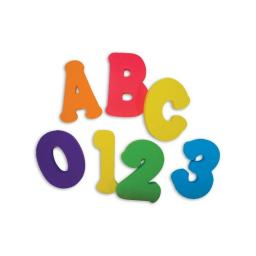# Determine 2944

Determine an unknown number. Its double is equal to its triple less 1.5.

x =  1.5

### Step-by-step explanation:

2x = 3x - 1.5

2·x = 3·x - 1.5

x = 1.5

x = 1.5/1 = 1.5

x = 3/2 = 1.5

Our simple equation calculator calculates it.Did you find an error or inaccuracy? Feel free to write us. Thank you!

Tips for related online calculators
Do you have a linear equation or system of equations and looking for its solution? Or do you have a quadratic equation?